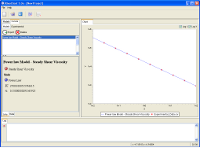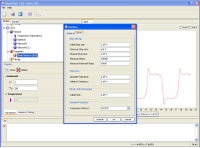RheoChart

RheoChart is a charting program to analyse the behaviour of a large range of differential viscoelastic models. In a few clicks, the response of models such as the Giesekus, PTT or the DCPP models can be analysed in a dynamic chart.

RheoChart also helps to determine the parameters of a model by fitting the model response to experimental data. The parameters calibration procedure is greatly simplified if not completely automatic.DCPP multi-mode model: main panel
Features

1) Rheological Modelling
• Generalised Newtonian models
• Newtonian (constant)
• Power law
• Bird-Carreau-Yasuda
• Cross
• Bingham
• Herschel-Bulkley
• Log-Log
• Multi-mode differential viscoelastic models
• Double Convected Pom-Pom (DCPP)
• Giesekus
• Phan Thien-Tanner (PTT)
• Upper-Convected Maxwell (UCM)
• Oldroyd-B
• FENE-P
• White-Metzner
• Temperature dependence
• No dependence
• Williams-Landel-Ferry (WLF)
• WLF shear stress
• Arrhenius
• Arrhenius shear stress
• Arrhenius Approximate
• Arrhenius Approximate shear stress
• Fulcher
2) Material Functions
• Linear Properties
• Shear Storage Modulus
• Shear Loss Modulus
• First Normal Stress Coefficient
• Second Normal Stress Coefficient
• Transient Shear Flow
• Shear Stress Growth coefficient
• First Normal Stress Growth coefficient
• Second Normal Stress Growth coefficient
• Large Amplitude Oscillatory Shear stress (LAOS)
• Multi-Ramp Shear stress: arbitrary multi-ramp function of the shear rate
• Transient Extensional Flow
• Tensile Stress Growth coefficient
• Biaxial Stress Growth coefficient
• Planar Stress Growth coefficient
3) Automatic Fitting - Parameters Calibration

RheoChart has the unique capability to fit model predictions against experimental data. This procedure sometimes referred as parameters calibration can be very tedious to accomplish manually. RheoChart can greatly simplify this procedure.

All parameters of a model (Generalised Newtonian models or Multi-mode differential viscoelastic models) can be estimated using several optimisation algorithm. These parameters can be bound by upper and/or lower-limits or left to vary freely. Alternatively, parameters can be fixed.

Every material functions available in RheoChart can be used to curve fit experimental data. A fitting procedure can use one or more experimental curves simultaneously. A weight can be associated with each experimental curve.

4) Graphical Interface
• Project
• Multiple models can be defined in a project
• Multiple Fittings can be defined for each models
• Dynamic Charts
• Zoom/Unzoom using the mouse
• Switch between linear and logarithm scales
• Tabbed charts (several charts can be created simultaneously)
• Charts can be saved as images or copied to the clipboard
• Curves
• Model predictions can be exported in CSV files
• Experimental curves can be imported in CSV format
• Experimental points can be edited/deletedPower Law model: curves panelGeneral Settings Dialog